Function Repository Resource:

# MappedTransformedDistribution

Transform a statistical distribution by applying the same function to all of its arguments

Contributed by: Isaac Chandler and Seth J. Chandler
 ResourceFunction["MappedTransformedDistribution"][f,dist] creates a transformed distribution for which the function f is mapped over all of the arguments.

## Details and Options

If the distribution has just one argument, the function f is applied to that argument and not "mapped" over it.

## Examples

### Basic Examples (3)

Compute the CDF of a product distribution of two binomial distributions in which 1 has been added to each of the arguments:

 In:=Out=Compute the CDF of a lognormal distribution in which the argument has been negated:

 In:=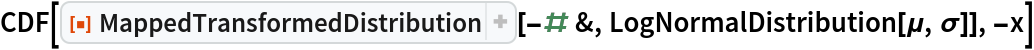Out=Compute the SurvivalFunction of a binomial distribution in which the square root is applied to its argument:

 In:=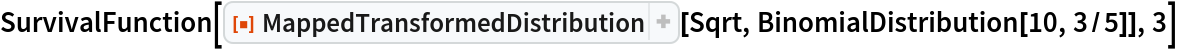Out=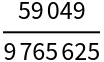### Scope (3)

Compute the mean of a mapped transformed distribution of a multivariate discrete distribution:

 In:=Out=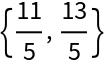Compute the characteristic function of a mapped transformed distribution of a multivariate symbolic continuous distribution:

 In:=Out=Compute the mean of a mapped transformed distribution of a copula distribution of two binomial distributions:

 In:=Out=### Applications (1)

Plot the CDF of a copula distribution whose components are binomial and each argument of which has been transformed by adding 1 to it:

 In:=Out=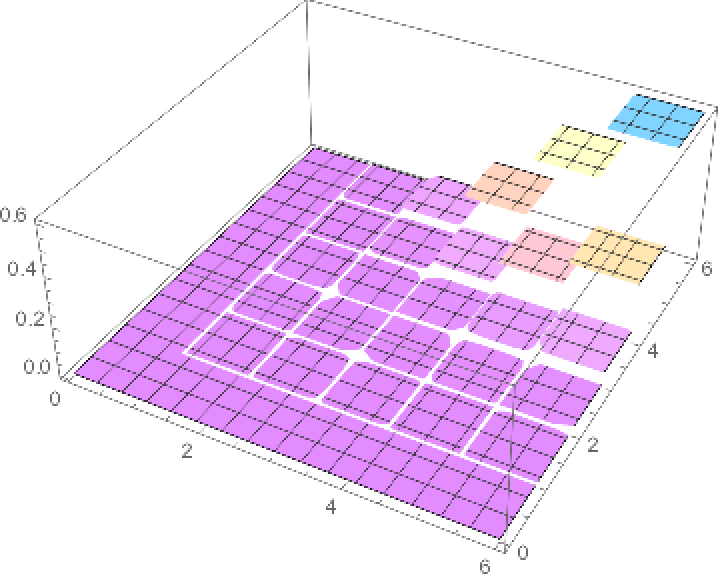### Properties and Relations (2)

If the function mapped over the arguments to a continuous distribution is negation, that is, -#&, the roles of CDF and SurvivalFunction are effectively exchanged:

 In:=Out=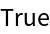The SurvivalFunction of a discrete distribution evaluated at x is the same as the CDF of the mapped transform distribution evaluated at -(x+1), where the mapped function is -#& and the distribution is defined over integers:

 In:=Out=### Neat Examples (1)

Compute the probability that a person alive at age 61 will be dead before age 71 given that their mortality is determined by a discretized variant of a Gompertz–Makeham mortality function:

 In:=In:=Out=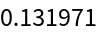Seth J. Chandler

## Version History

• 1.0.0 – 31 July 2019